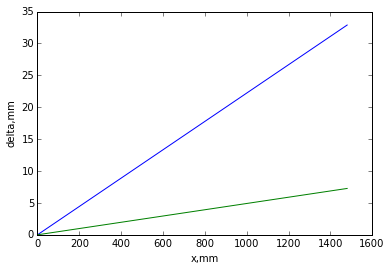# Chapter 10:Approximate Solutions of the Navier-Stokes Equation¶

## Example 10.10-2, Page No:499¶

In :
import math

#Variable Decleration
rho_air=0.8588 #Density of the surrounding air by gas law in kg/m^3
u=1.474*10**-5 #Dynamic Viscosity in kg/m.s
rho_particle=1240 #Density of the particle in kg/m^3
D=50*10**-6 #Diameter of the particles in m
g=9.81 #Acceleration due to gravity in m/s^2

#Calculations
#Applying Newtons Third Law and solving for V we get
V=(D**2/(18*u))*(rho_particle-rho_air)*g #Terminal Velocity of the particle in m/s

#Verification of Reynolds Number
Re=(rho_air*V*D)/u #Reynolds Number

#Result
print "The Terminal Velocity of the particles is",round(V,3),"m/s"

The Terminal Velocity of the particles is 0.115 m/s


## Example 10.10-6,Page No:519¶

In :
import math

#Variable Decleration
V_dot_by_L1=2 #Strength of source in line 1 in m^2/s at (0,-1)m
V_dot_by_L2=-1 #Strength of source in line 2 in m^2/s at (1,-1)m
T=1.5 #Vortex Strength in m^2/s a (1,1) m
r_vortex=1 #distance in m
r_source1=1.414 #Distance in m
r_source2=1 #Distance in m

#Calculations
V_vortex=T/(2*pi*r_vortex) #Velocity Induced by the Vortex in m/s
V_source1=V_dot_by_L1/(2*pi*r_source1) #Velocity induced by the source1 in m/s
V_source2=V_dot_by_L2/(2*pi*r_source2) #Velocity induced by the source2 in m/s
V=V_vortex+(V_source1/2**0.5)+V_source2+(V_source1/2**0.5) #Vectorial addition of the velocities in m/s

#Result
print "The superposed velocity at point (1,0) is",round(V,3),"m/s to the right"

The superposed velocity at point (1,0) is 0.398 m/s to the right


## Example 10.10-8, Page No:526¶

In :
import math

#Variable Decleration
V_dot=0.11 #Volumetric flow rate in m^3/s
L=0.35 #Length of flow in m
b=0.02 #m
u_star_max=0.159 #m/s

#Calculations
V_dot_by_L=-V_dot/L #Strength of the line source in m^2/s
u_max=(-u_star_max*V_dot_by_L)/b #Umax in m/s

#Result
print "The strength of the line source is",round(V_dot_by_L,3),"m^2/s"
print "The maximum velocity is",round(u_max,2),"m/s"

The strength of the line source is -0.314 m^2/s
The maximum velocity is 2.5 m/s


## Example 10.10-9,Page No:535¶

In :
import math

#Variable Decleration
V=10 #Velocity of fluid Flow in kh/hr
rho=999.9 #Density of water at 5˚C in kg/m^3
v=1.519*10**-6 #Kinematic Viscosity in m^2/s
#Conversion Factors
C1=1000
C2=3600

#Calculations
Rex=(V*0.5*C1)/(v*C2) #Reynolds Number

#Result
print "The Reynolds Number is",round(Rex)
print "As a result the boundary layer is Transitional"

The Reynolds Number is 914344.0
As a result the boundary layer is Transitional


## Example 10.10-10,Page No:541¶

In :
import math

#Variable Decleration
V=32 #Velocity of the fluid in m/s
x=1.1 #Distance in m
v=1.7*10**-5 #Kinematic Viscosity in m^2/s
#Conversion factor
C1=1000
C2=3600
C3=100

#Calculations
Rex=(V*x*C1)/(v*C2) #Reynolds Number
delta=4.91*x*C3/Rex**0.5 #Thickness of boundary Layer in cm

#Result
print "The Thickness of the boundary Layer is",round(delta,2),"cm"

The Thickness of the boundary Layer is 0.71 cm


## Example 10.10-11, Page No:546¶

In :
import math

#Variable decleration
x=0.3 #Length of the tunnel in m
V=4 #Optimised speed in m/s
R=0.15 #Radius of the tunnel in m
v=1.507*10**-5 #Kinematic Viscosity in m^2/s

#Calculations
Rex=(V*x)/v #Reynolds Number
#Using the diplacement thickness formula
delta_star=(1.72*x)/Rex**0.5 #Displacement Thickness in m
#Applying the Continuity Equation
V_end=(V*R**2)/(R-delta_star)**2 #Velocity at the end in m/s

#Result
print "The end velocity should be",round(V_end,2),"m/s"

The end velocity should be 4.1 m/s


## Example 10.10-12, Page No:550¶

In :
import math
import matplotlib.pyplot as plt
%matplotlib inline

#NOTE:The Notation has been changed here

#Decleration of variables
V=10 #Velocity in m/s
x=1.52 #Length in m
v=1.516*10**-5 #Kinematic viscosity in m^2/s
u=[0,0.]
#Calculations
#Part(a)

length=range(0,1500,20) #Array of length in mm
Re=(V*x)/v #Reynolds Number
y=transpose(length) #Transpose of the length matrix
#Laminar Boundary Layer
d_lam=(4.918*y)/((Re)**0.5)
#Turbulent Boundary Layer
d_tur=(0.16*y)/((Re)**(0.14285))

#Part(b)
C_lam=0.664/((Re)**0.5) #Local Skin Friction coefficient for laminar boundary layer
C_tur=0.027/((Re)**0.14285) #Local Skin Friction coefficient for turbulent boundary Layer

#Result
print "The Reynolds Number is",round(Re)
print "The Local skin friction coefficient is",round(C_lam,4),"for laminar boundary layer"
print "The Local Skin friction coefficient is",round(C_tur,4),"for turbulent boundary layer"

plt.plot(y,d_tur,y,d_lam)
plt.xlabel('x,mm')
plt.ylabel('delta,mm')
plt.show()

The Reynolds Number is 1002639.0
The Local skin friction coefficient is 0.0007 for laminar boundary layer
The Local Skin friction coefficient is 0.0038 for turbulent boundary layer## Examples 10.10-15,Page No:563¶

In :
import math

#Variable Decleration
rho=1.204 #Density of the fluid in kg/m^3
w=0.5 #Width of the test object in m
U=10 #Velocity of the flow in m/s
delta1=0.042 #Boundary Layer thickness at 1 in m
delta2=0.077 #Boundary Layer thickness at 2 in m

#Calculations
a=(delta2-delta1)
b=w*rho
c=U**2
Fd=(b*c*a*4)/45#Skin friction drag in N

#Result
print "The Skin Friction Drag is",round(Fd,2),"N"

The Skin Friction Drag is 0.19 N